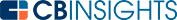# What is an Inventory Turnover Ratio?

An inventory turnover ratio is a calculated measurement of sales of inventory over a given period of time. It is also known as inventory turns or stock turnover. A business’s inventory turnover ratio reveals the speed at which its entire inventory is sold and replenished.

A business can use its inventory turnover ratio to assess the number of sales of specific products or product groups in a week, month, or year.

The most common use of this ratio, however, is to measure a company’s entire inventory sales rate at once. Inventory turnover ratio is also useful for tracking sales performance.

## What different ratios mean

A balanced inventory turnover ratio is usually ideal. A high inventory turnover ratio may highlight issues with supply and insufficient inventory to meet demand, while a low ratio could reveal a sales slump or overstocking. Findings can reveal issues with inventory management or the need to adapt to market fluctuations. What constitutes a high or low ratio depends on the industry and a given company's sales performance history.

The way inventory turnover ratios are calculated determines the result. A special formula is selected and used consistently to provide measured insight into a business's inventory turnover.

## Types of inventory turnover ratio formulas (example included)

There are multiple formulas that can be used to calculate inventory turnover ratio. The following can both be used for this purpose, though their results may vary:

• Inventory turnover = net sales / average inventory at selling price
• Inventory turnover = cost of goods sold (COGS) / average inventory at cost

Average inventory is a useful metric needed to calculate inventory turnover ratios in both of the formulas above. It can be used to track both product quantities and values over time. In the two formulas above, average inventory is calculated based on the final sales prices of products or their production costs.

The formula for average inventory is as follows:

Beginning inventory + ending inventory / number of time periods (in this case, there would be two)

## Inventory turnover ratio example

To use a practical example, let’s calculate a theoretical company’s inventory turnover ratio using the second formula above. We based our calculation on the following assumptions:

• \$6.6M = the company’s net sales for the year
• \$9.2M = value of the company’s beginning inventory at selling price
• \$9.4M = value of the company’s ending inventory at selling price

We’ll call this hypothetical company ABC Company, an enterprise organization in the consumer healthcare products business. According to this data, ABC’s inventory turnover ratio can be calculated by the following formula:

6.6M

━━━━━━━━━━

(9.2M + 9.4M) / 2

This yields a result of 0.71, meaning that ABC Company sold 71% of its product inventory in the year.

In this example, ABC Company’s inventory turnover ratio is fairly low. By implication, the company's products remain in stock for a longer amount of time before being sold. This result can still be considered reasonably good for a sizable organization with a breadth of products across multiple niches.

ABC Company’s inventory turnover ratio would not be as acceptable for a company that offers products meant for immediate consumption. A higher ratio would match the increased speed at which such products are intended to be sold.

Higher ratios can be a positive indication of a business's performance. However, it is important to first compare inventory turnover ratios of other similar firms in a given sector to determine what the average is. This average can then be used as a baseline for future analyses.

An inventory turnover ratio is a useful calculation for businesses to refer to. It can provide them with a number of insights regarding their inventory management, specifically in relation to how efficiently it is being managed.

You may also like# www.cbinsights.com